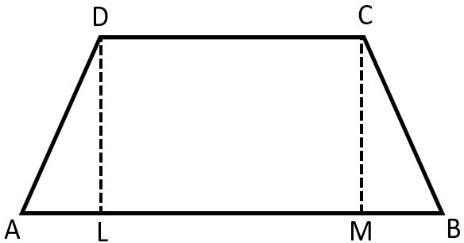QUESTION

# The perimeter of an isosceles trapezium is $16cm$ and the shorter parallel side is one third of the longer parallel side. If the sum of the lengths of the opposite sides of the trapezium are equal, find the area (in $c{{m}^{2}}$) of the trapezium?    (A) $8\sqrt{3}$    (B) $4\sqrt{3}$    (C) $12\sqrt{3}$    (D) $16\sqrt{3}$

Hint: Here, first we have to draw the figure. Draw $DL\bot AB$ and $CM\bot AB$ such that CD = ML and AL = MB. Here, we have to apply the formulas:
Perimeter of a trapezium = sum of its sides
Area of a trapezium = $\dfrac{1}{2}\times$sum of the parallel sides $\times$ height
$A{{D}^{2}}=A{{L}^{2}}+D{{L}^{2}}$

Complete step-by-step solution -
First, we have to draw the figure. Draw $DL\bot AB$ and $CM\bot AB$.Here we have to find the area of the trapezium.
We are given that the trapezium is isosceles, which means the opposite sides are equal. Therefore, we can say that:
We have the perimeter of the trapezium = $16cm$
Here, in trapezium we have $AB\parallel CD$.
We are also given that the shorter parallel side is one-third of the longer parallel side. i.e.
Now, consider the length of CD to be a. Then the length of AB will be 3 times that of CD.
CD = a ….. (2)
AB = 3a …. (3)
Hence, we are given that the lengths of the opposite sides are equal. We can write:
AD + BC = CD + AB
Hence, from equations (1) and equation (2), we can write:
By cross multiplication we get:
AD = $\dfrac{4}{2}a$
Now, by cancellation we obtain:
Therefore, we will obtain:
We know that the perimeter of a trapezium is the length of its four sides. i.e.
Perimeter = AB + BC + CD + AD
Now by substituting all the values we obtain:
16 = 3a + 2a + a + 2a
16 = 8a
By cross multiplication, we get:
$\dfrac{16}{8}$ = a
Now, by cancellation we obtain:
2 = a
Therefore the sides of the trapezium are:
CD = 2 cm
AD = $2\times 2$
BC = 4 cm
AB = 3a
AB= $3\times 2$
AB = 6 cm
Now, consider the right angled triangle, $\Delta ALD$, Hence by Pythagoras theorem, we have
$A{{D}^{2}}=A{{L}^{2}}+D{{L}^{2}}$
First we have to find AL.
From the figure, we can say that:
CD = ML =2 cm
AL = MB
Now, we can write:
AL + ML + MB = 6
2AL + 2 = 6
2AL = 4
By cross multiplication we get:
AL = $\dfrac{4}{2}$
By cancellation we get:
AL = 2 cm
We have AD = 4cm, AL = 2 cm. Now we have to find DL using Pythagoras theorem. i.e. we have:
\begin{align} & D{{L}^{2}}=A{{D}^{2}}-A{{L}^{2}} \\ & D{{L}^{2}}={{4}^{2}}-{{2}^{\begin{smallmatrix} 2 \\ \end{smallmatrix}}} \\ & D{{L}^{2}}=16-4 \\ & D{{L}^{2}}=12 \\ \end{align}
Now, by taking square root on both the sides we get:
\begin{align} & \sqrt{D{{L}^{2}}}=\sqrt{12} \\ & DL=\sqrt{3\times 2\times 2} \\ & DL=2\sqrt{3} \\ \end{align}
Therefore, we will get DL = $2\sqrt{3}$ cm.
Next, we have to find the area of the trapezium, we know that:
Area of the trapezium = $\dfrac{1}{2}\times$sum of the parallel sides $\times$ height
Hence, we have the parallel sides as AB and CD and the height is AL
\begin{align} & Area=\dfrac{1}{2}\times (AB+CD)\times AL \\ & Area=\dfrac{1}{2}\times (6+2)\times 2\sqrt{3} \\ & Area=\dfrac{1}{2}\times 8\times 2\sqrt{3} \\ & Area=\dfrac{16\sqrt{3}}{2} \\ \end{align}
Now by cancellation we obtain:
$Area=8\sqrt{3}c{{m}^{2}}$. Hence the required area of the trapezium is $8\sqrt{3}c{{m}^{2}}$.
Therefore, the correct answer for this question is option (a).

Note: Here we have two right angled triangles $\Delta ALD$ and $\Delta BMC$, DL and CM are the heights where, DL = CM. So instead of $\Delta ALD$ you can also consider $\Delta BMC$ to find height CM.